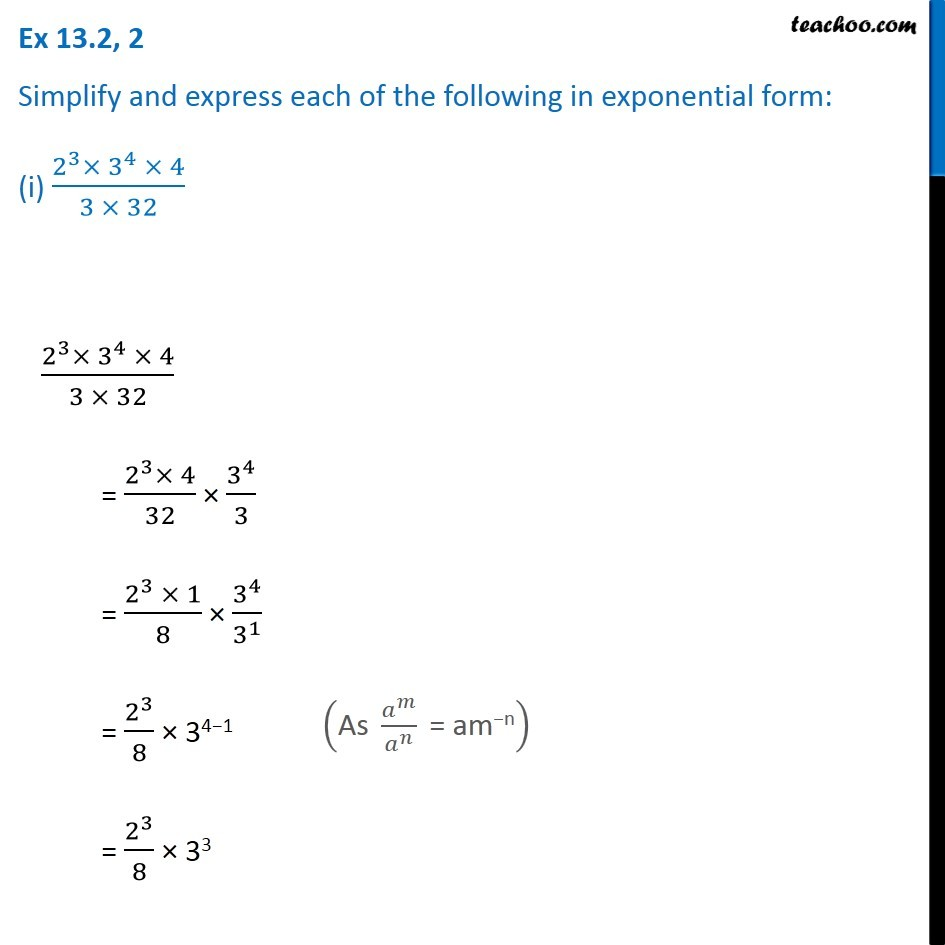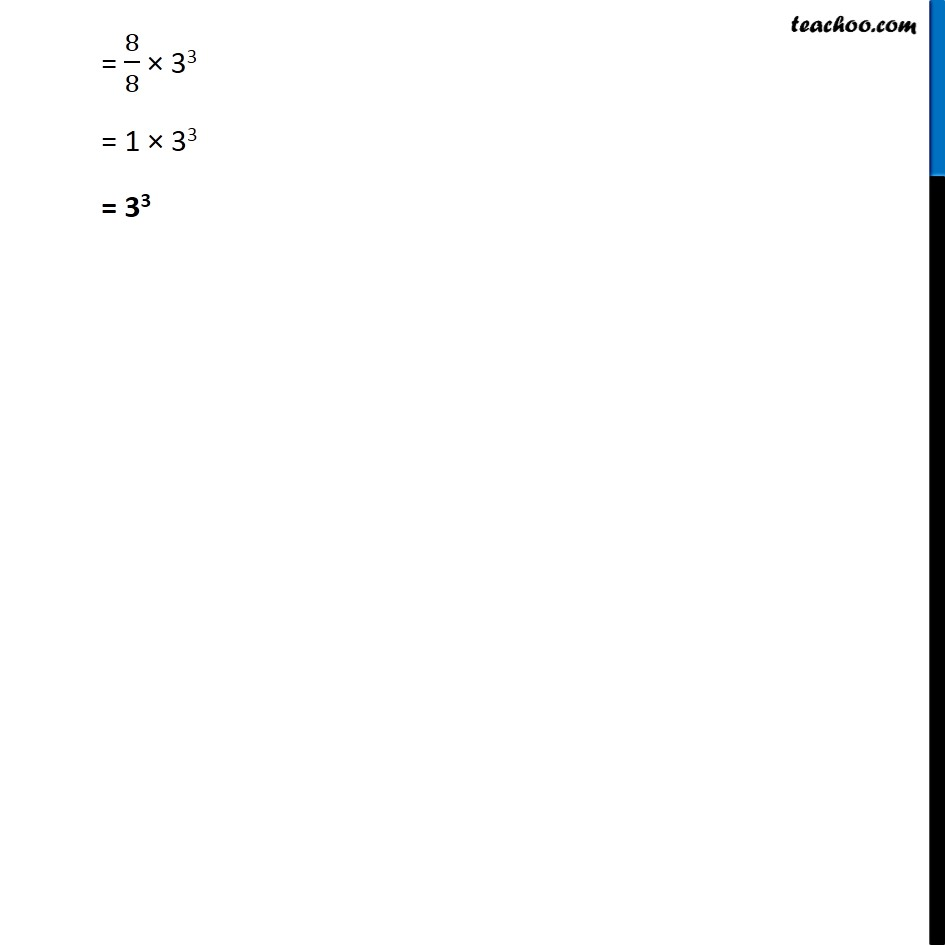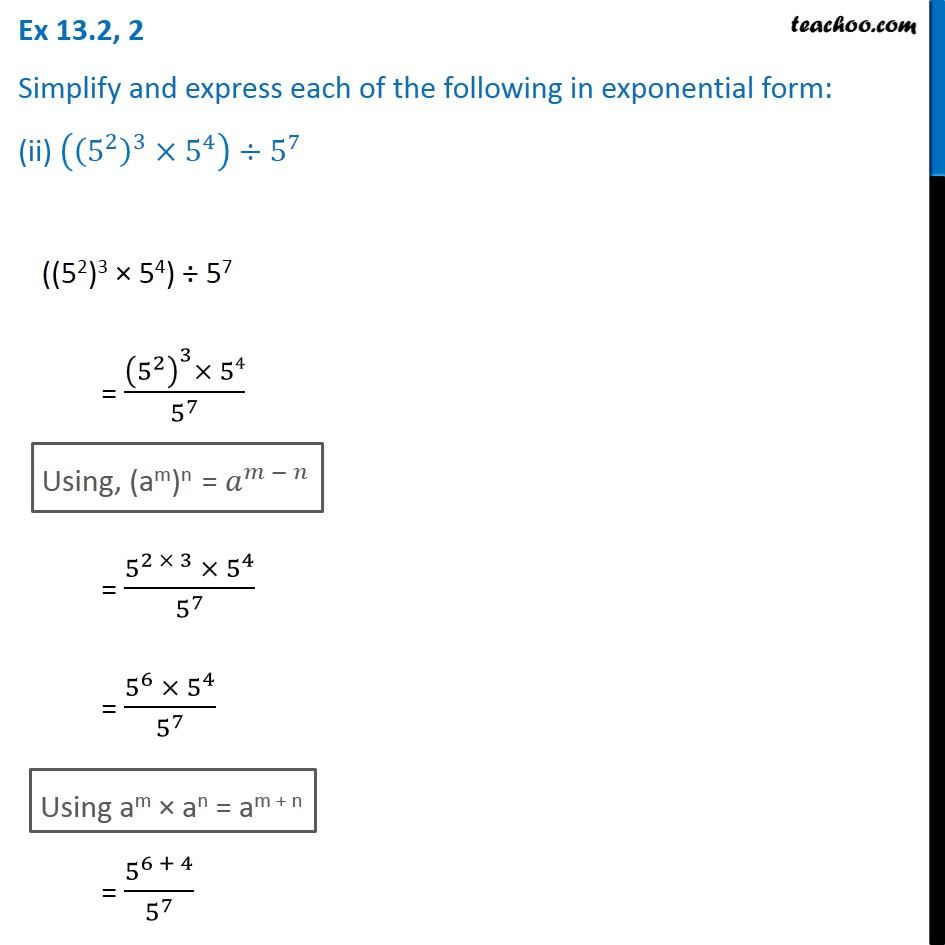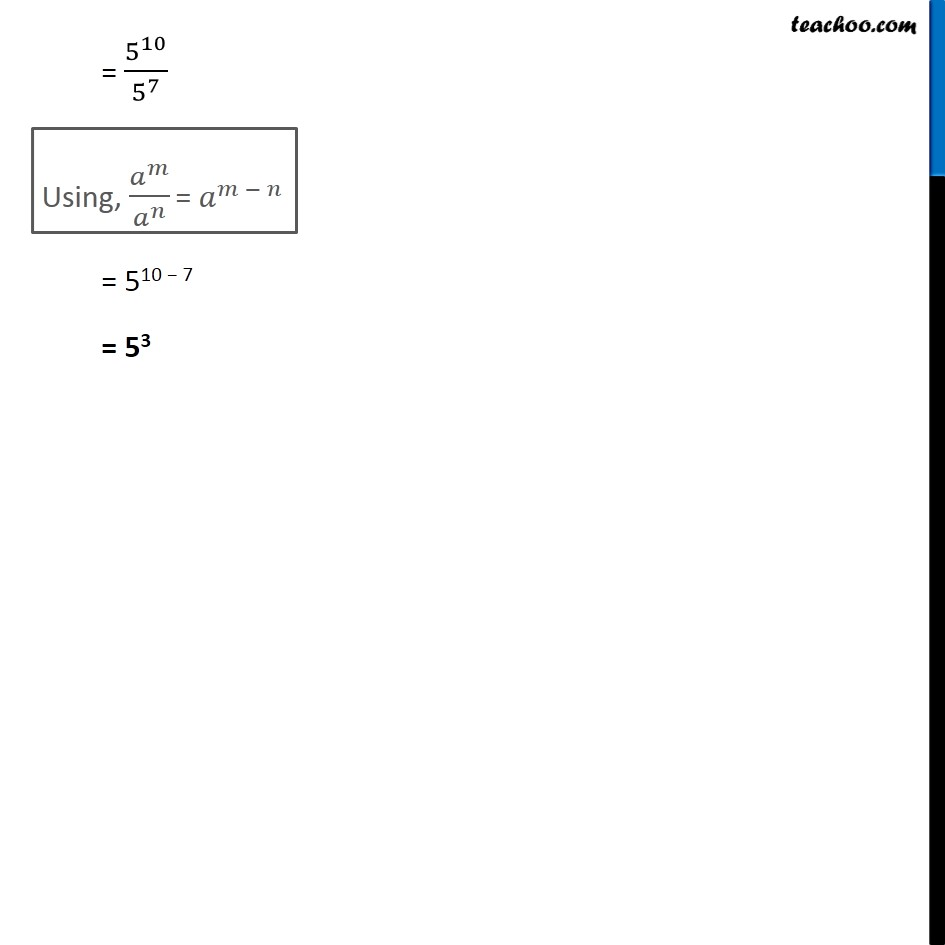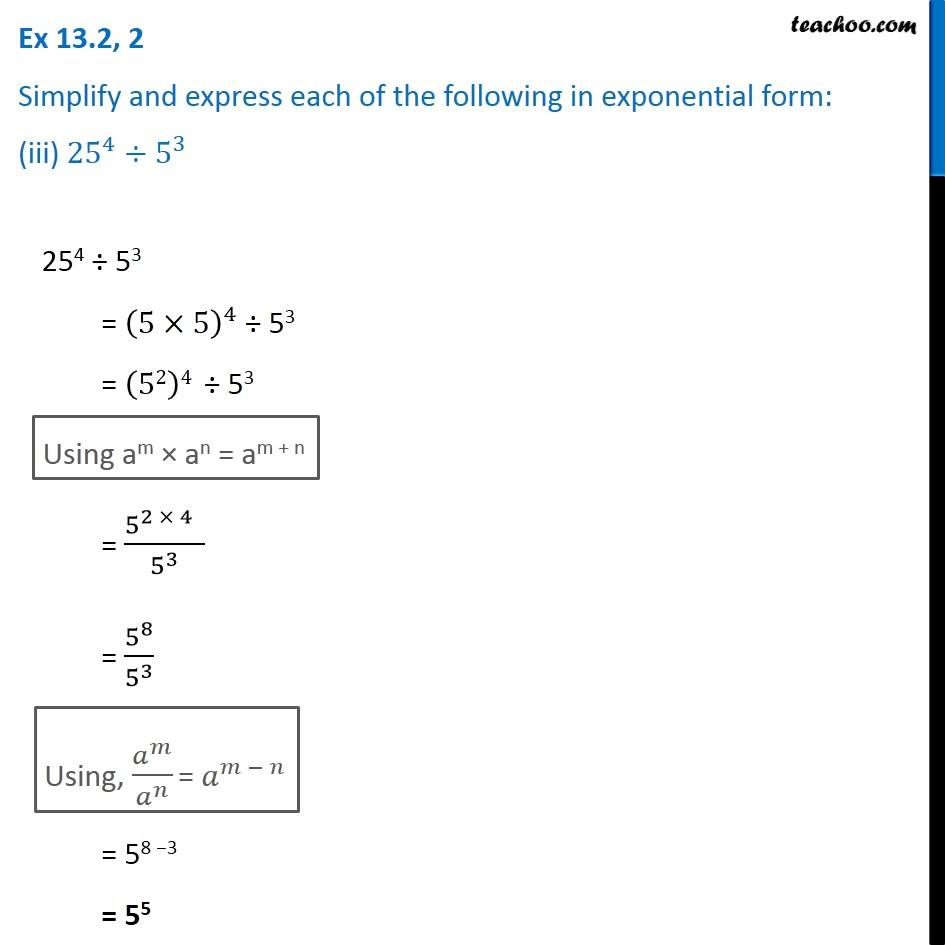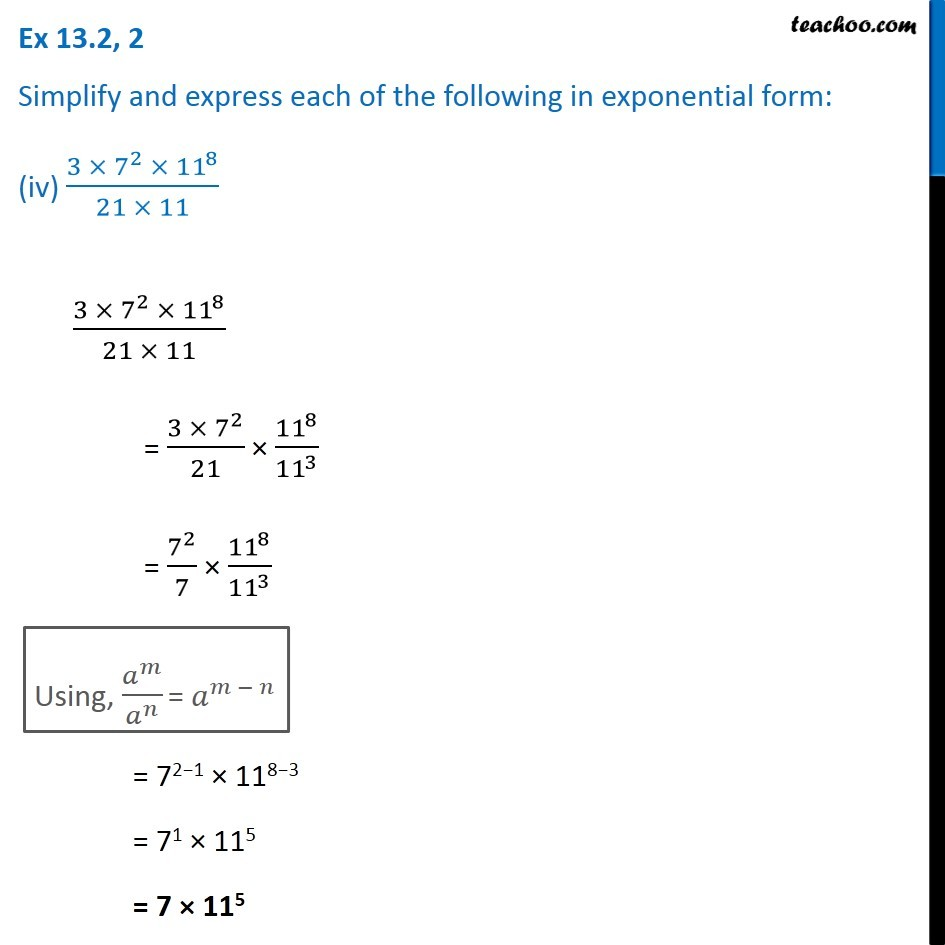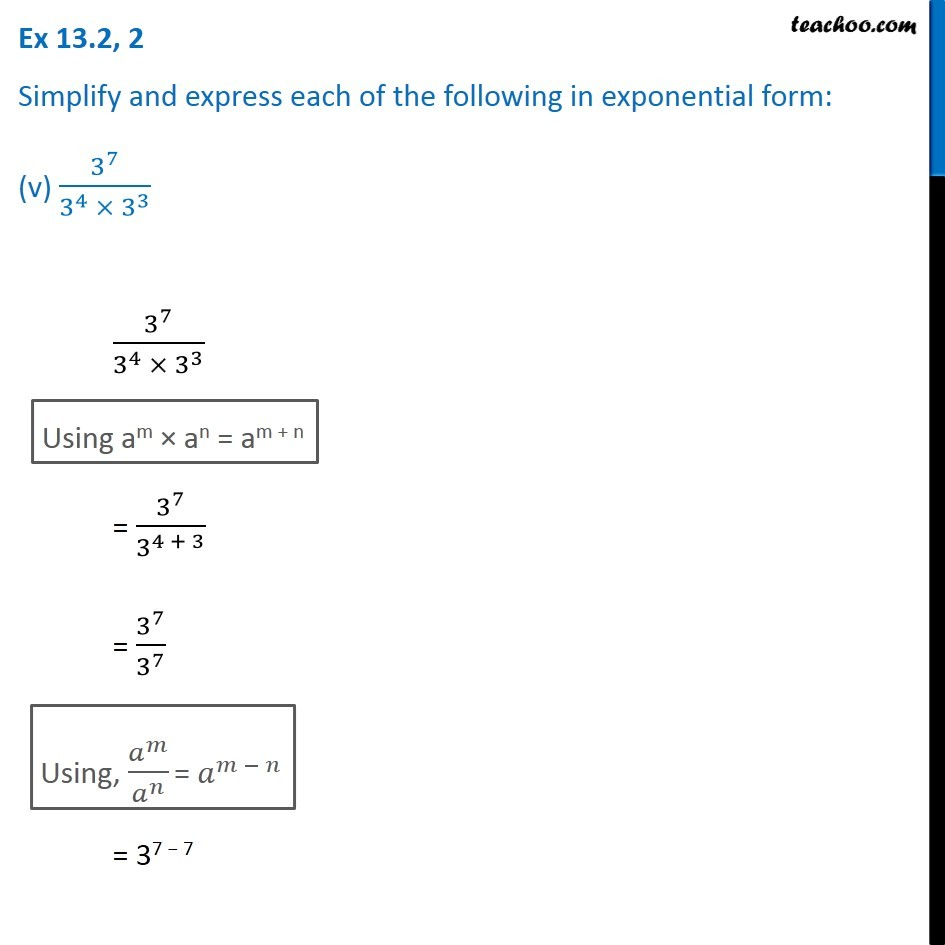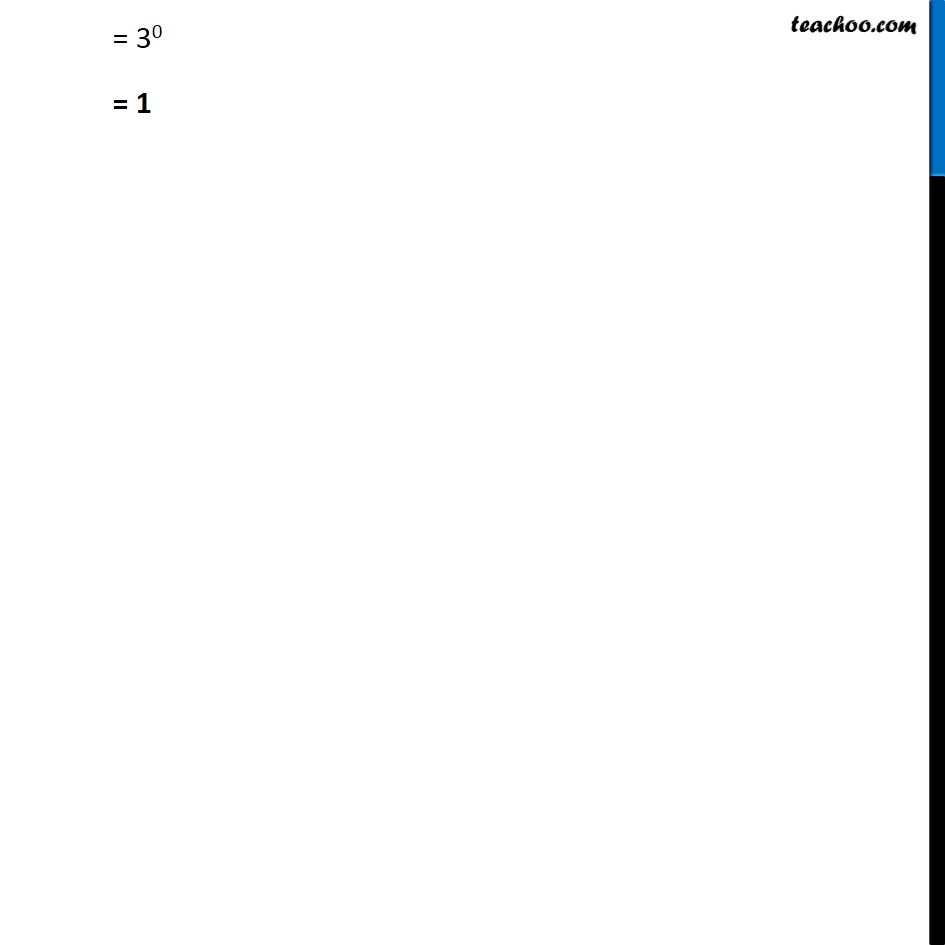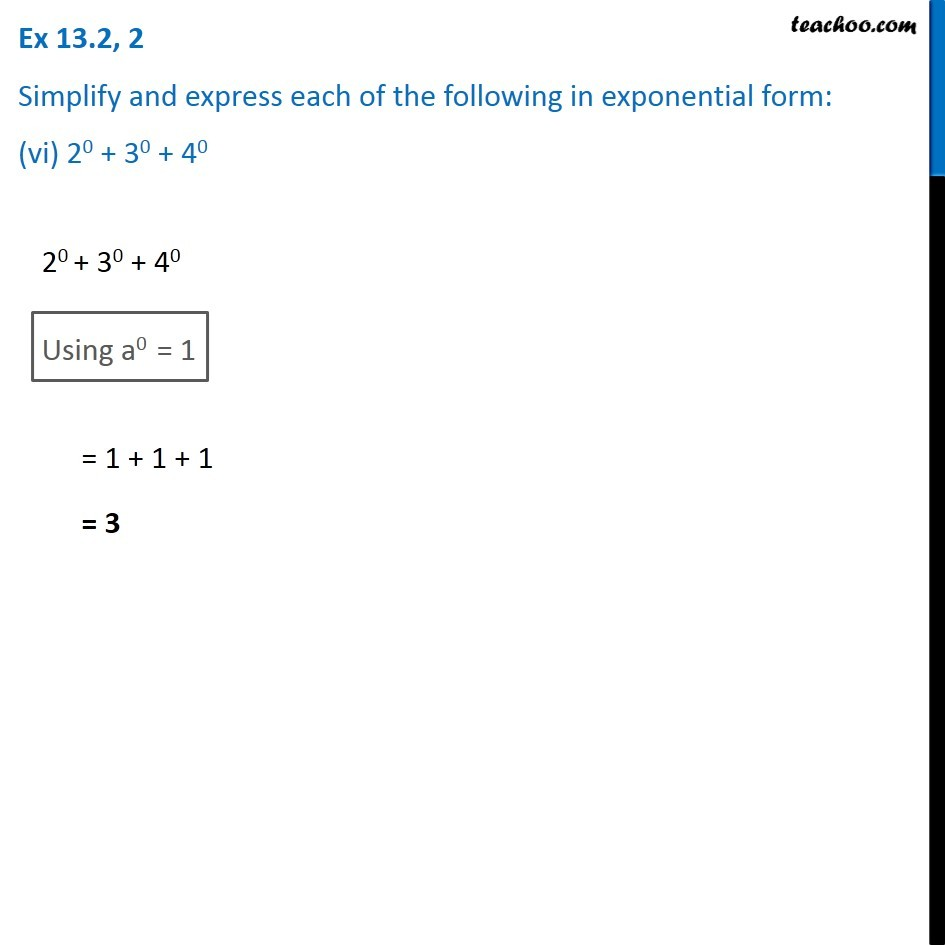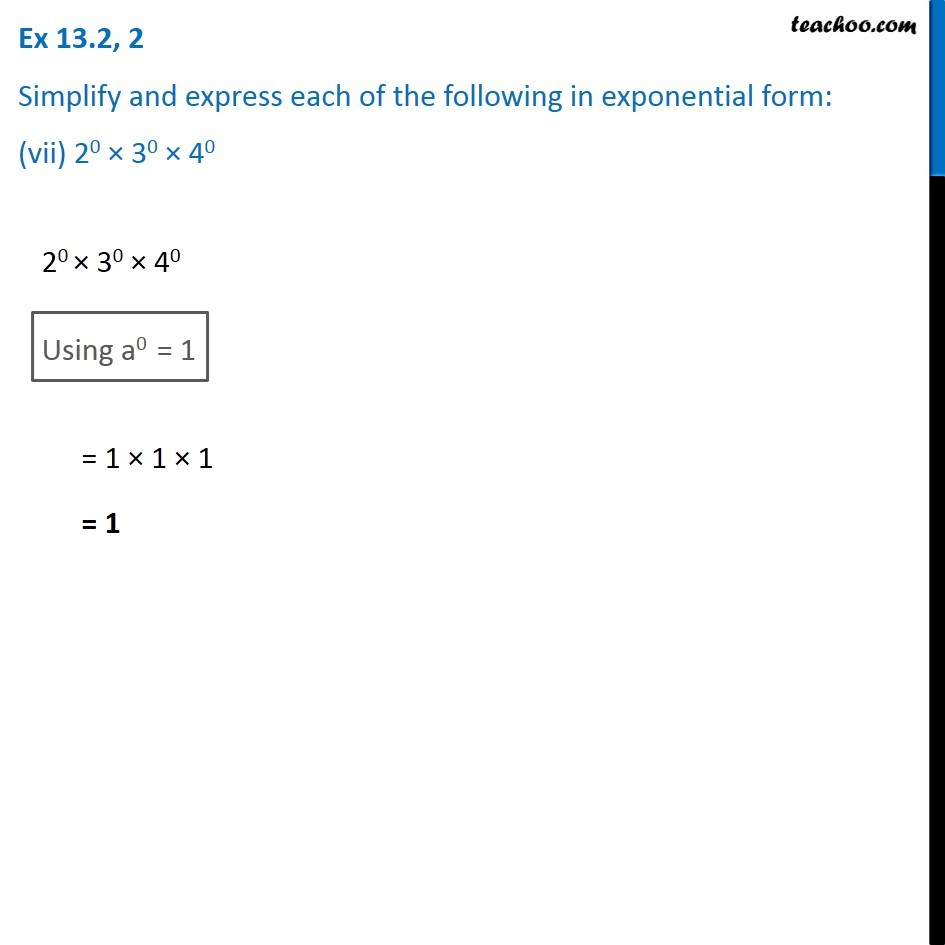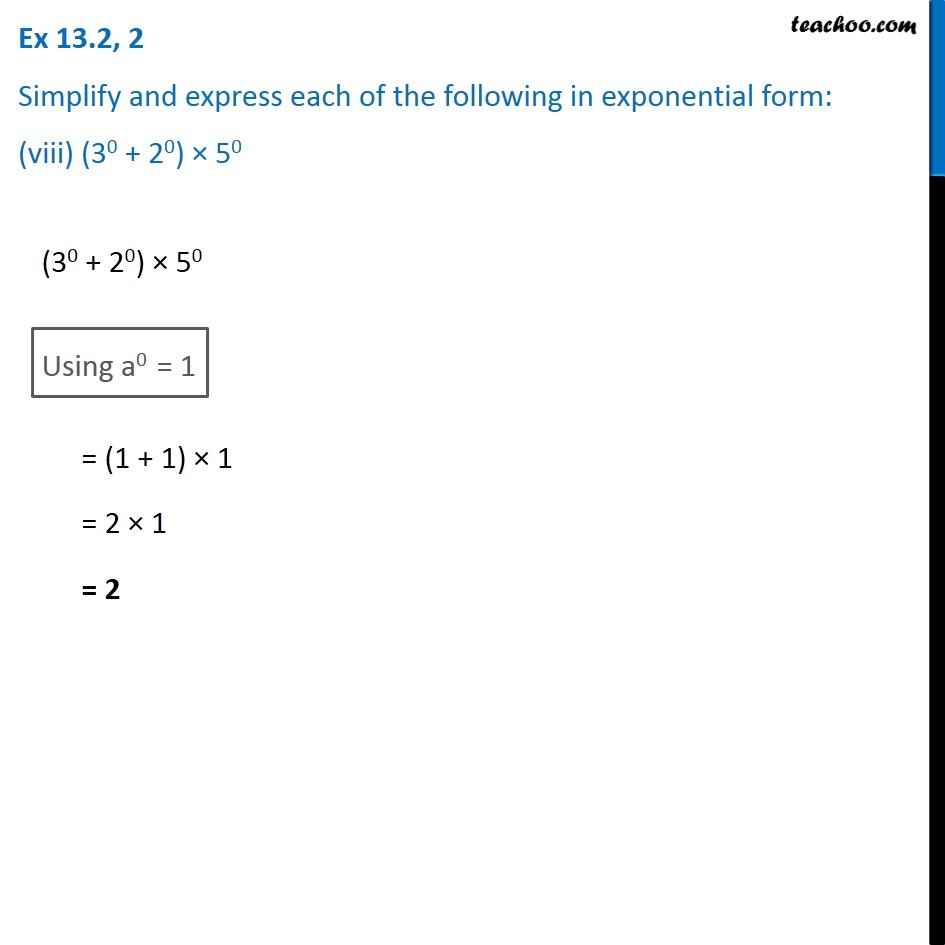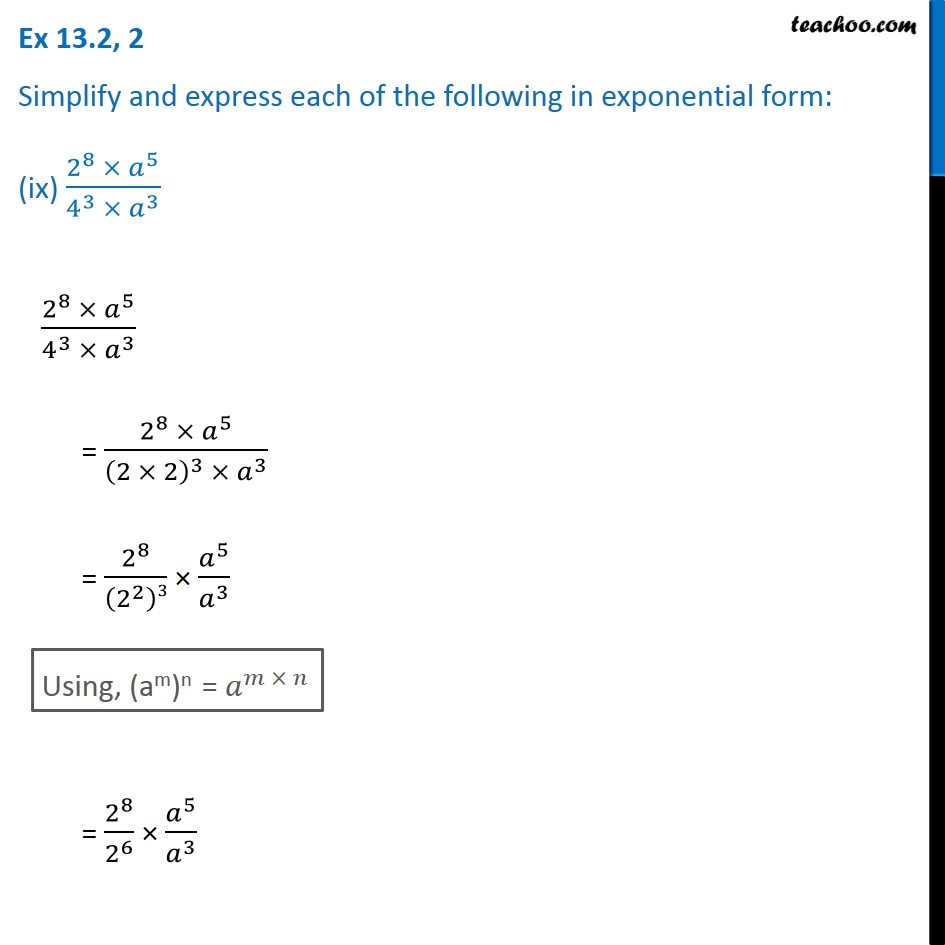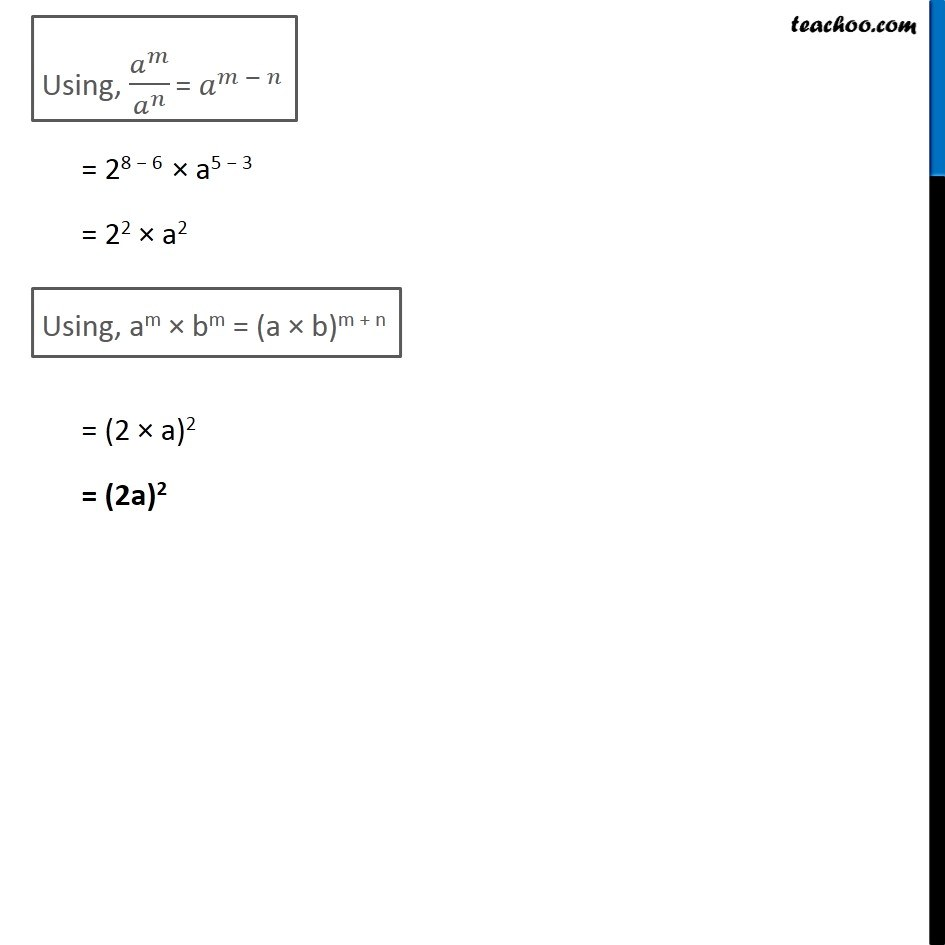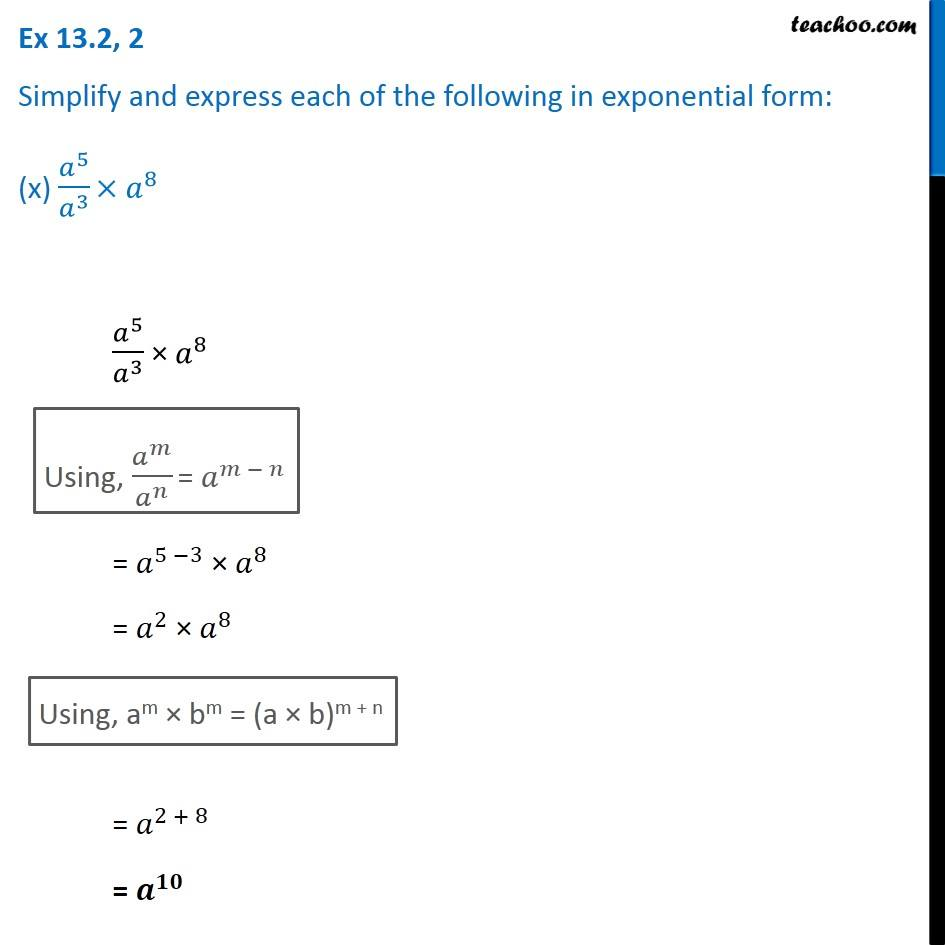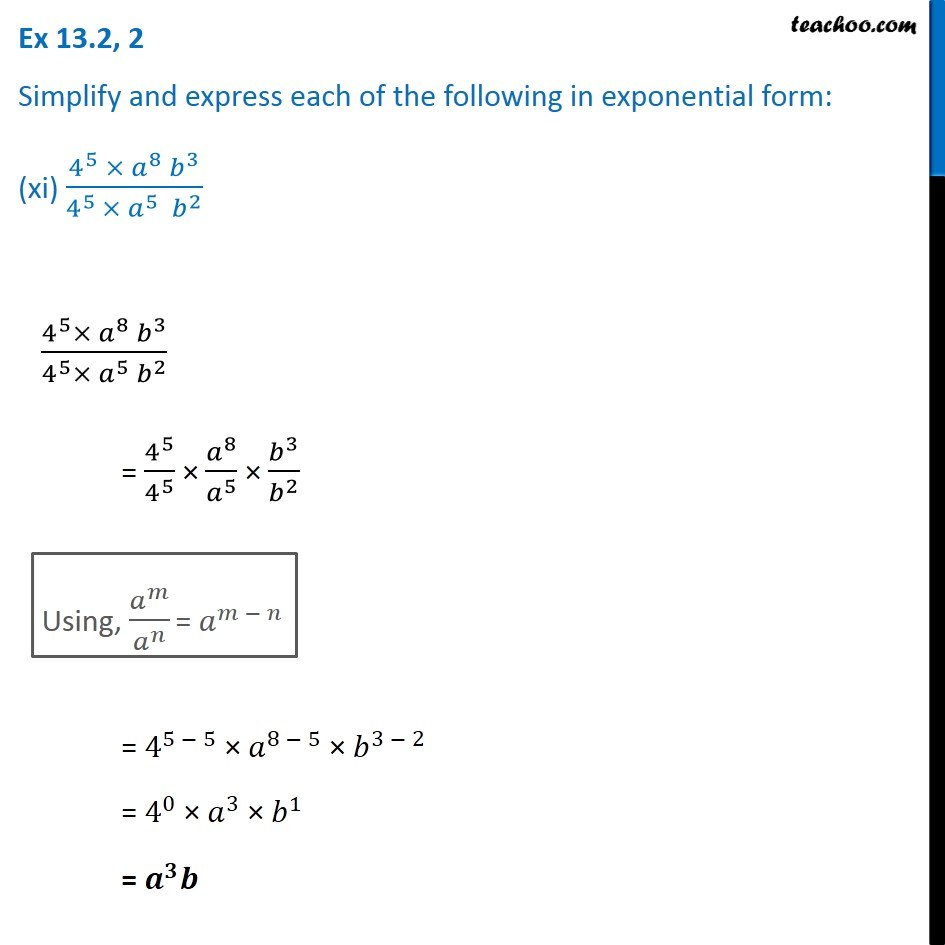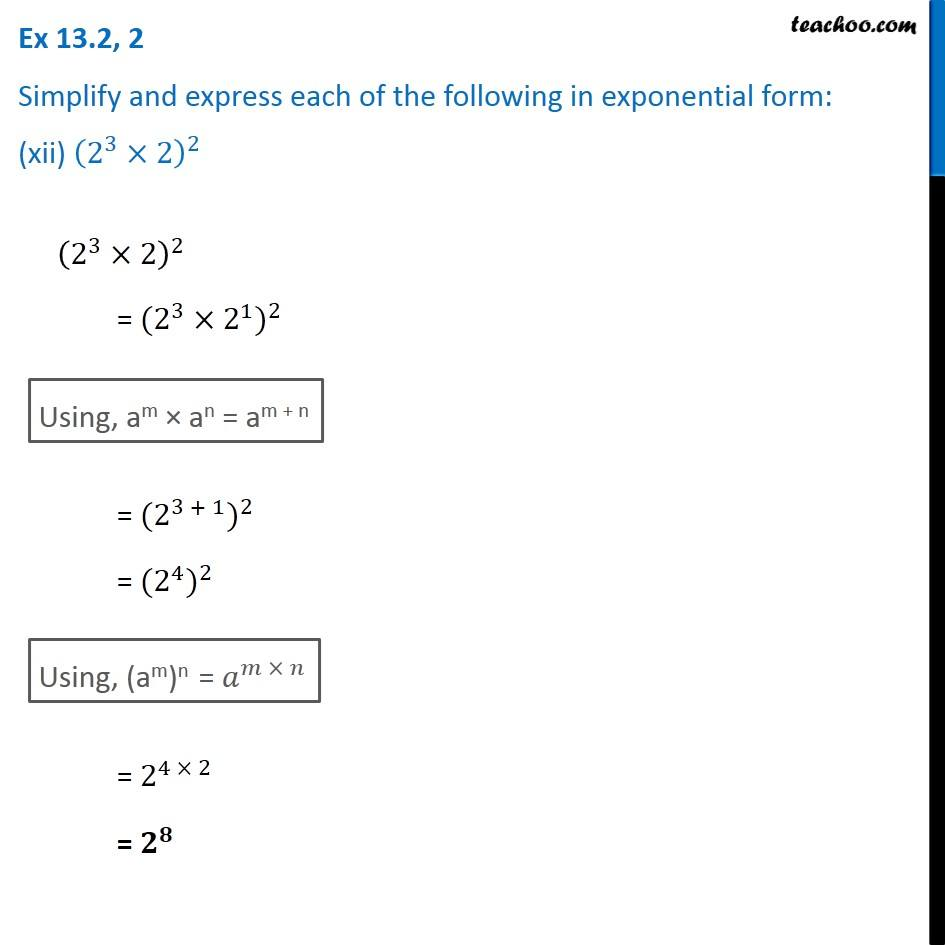1. Chapter 13 Class 7 Exponents and Powers
2. Serial order wise
3. Ex 13.2

Transcript

Ex 13.2, 2 Simplify and express each of the following in exponential form: (i) (2^3× 3^4 × 4)/(3 × 32) (2^3× 3^4 × 4)/(3 × 32) = (2^3× 4)/32 × 3^4/3 = (2^3 × 1)/8 × 3^4/3^1 = 2^3/8 × 34−1 = 2^3/8 × 33 ("As " 𝑎^𝑚/𝑎^𝑛 " = am−n" ) = 8/8 × 33 = 1 × 33 = 33 Ex 13.2, 2 Simplify and express each of the following in exponential form: (ii) ((5^2 )^3×5^4 )÷5^7 ((52)3 × 54) ÷ 57 = ((5^2 )^3× 54)/5^7 Using, (am)n = 𝑎^(𝑚 − 𝑛) = (5^(2 × 3) × 5^4)/5^7 = (5^6 × 5^4)/5^7 Using am × an = am + n = 5^(6 + 4)/5^7 = 5^10/5^7 Using, 𝑎^𝑚/𝑎^𝑛 = 𝑎^(𝑚 − 𝑛) = 510 − 7 = 53 Ex 13.2, 2 Simplify and express each of the following in exponential form: (iii) 〖25〗^4÷5^3 254 ÷ 53 = (5×5)^4 ÷ 53 = (52)4 ÷ 53 Using am × an = am + n Using am × an = am + n Using, 𝑎^𝑚/𝑎^𝑛 = 𝑎^(𝑚 − 𝑛) = 58 −3 = 55 Ex 13.2, 2 Simplify and express each of the following in exponential form: (iv) (3 ×〖 7〗^2 ×〖 11〗^8)/(21 × 11) (3 ×〖 7〗^2 ×〖 11〗^8)/(21 × 11) = (3 ×〖 7〗^2)/21 × 〖11〗^8/〖11〗^3 = 7^2/7 × 〖11〗^8/〖11〗^3 Using, 𝑎^𝑚/𝑎^𝑛 = 𝑎^(𝑚 − 𝑛) = 72−1 × 118−3 = 71 × 115 = 7 × 115 Ex 13.2, 2 Simplify and express each of the following in exponential form: (v) 3^7/(3^4 ×〖 3〗^3 ) 3^7/(3^4 ×〖 3〗^3 ) Using am × an = am + n = 3^7/3^(4 + 3) = 3^7/3^7 Using, 𝑎^𝑚/𝑎^𝑛 = 𝑎^(𝑚 − 𝑛) = 37 − 7 = 30 = 1 Ex 13.2, 2 Simplify and express each of the following in exponential form: (vi) 20 + 30 + 40 20 + 30 + 40 Using a0 = 1 = 1 + 1 + 1 = 3 Ex 13.2, 2 Simplify and express each of the following in exponential form: (vii) 20 × 30 × 40 20 × 30 × 40 Using a0 = 1 = 1 × 1 × 1 = 1 Ex 13.2, 2 Simplify and express each of the following in exponential form: (viii) (30 + 20) × 50 (30 + 20) × 50 Using a0 = 1 = (1 + 1) × 1 = 2 × 1 = 2 Ex 13.2, 2 Simplify and express each of the following in exponential form: (ix) (2^8 ×〖 𝑎〗^5)/(4^3 ×〖 𝑎〗^3 ) (2^8 × 𝑎^5)/(4^3 × 𝑎^3 ) = (2^8 ×〖 𝑎〗^5)/((2 × 2)^3 ×〖 𝑎〗^3 ) = 2^8/(2^2 )3 × 𝑎^5/𝑎^3 Using, (am)n = 𝑎^(𝑚 × 𝑛) = 2^8/2^6 × 𝑎^5/𝑎^3 Using, 𝑎^𝑚/𝑎^𝑛 = 𝑎^(𝑚 − 𝑛) = 28 − 6 × a5 − 3 = 22 × a2 Using, am × bm = (a × b)m + n = (2 × a)2 = (2a)2 = (2 × a)2 = (2a)2 Ex 13.2, 2 Simplify and express each of the following in exponential form: (x) 𝑎^5/𝑎^3 ×𝑎^8 𝑎^5/𝑎^3 × 𝑎^8 Using, 𝑎^𝑚/𝑎^𝑛 = 𝑎^(𝑚 − 𝑛) = 𝑎^(5 −3) × 𝑎^8 = 𝑎^2 × 𝑎^8 Using, am × bm = (a × b)m + n = 𝑎^(2 + 8) = 𝒂^𝟏𝟎 Ex 13.2, 2 Simplify and express each of the following in exponential form: (xi) (4^5 × 𝑎^8 𝑏^3)/(4^(5 )× 𝑎^5 𝑏^2 ) (4^5× 𝑎^8 𝑏^3)/(4^5×〖 𝑎〗^5 𝑏^2 ) = 4^5/4^5 × 𝑎^8/𝑎^5 × 𝑏^3/𝑏^2 Using, 𝑎^𝑚/𝑎^𝑛 = 𝑎^(𝑚 − 𝑛) = 4^(5 − 5) × 𝑎^(8 − 5) × 𝑏^(3 − 2) = 4^0 × 𝑎^3 × 𝑏^1 = 𝒂^𝟑 𝒃 Ex 13.2, 2 Simplify and express each of the following in exponential form: (xii) (2^3×2)^2 (2^3×2)^2 = 〖(2^3×2^1)〗^2 Using, am × an = am + n = 〖(2^(3 + 1))〗^2 = 〖(2^4)〗^2 Using, (am)n = 𝑎^(𝑚 × 𝑛) = 2^(4 × 2) = 𝟐^𝟖

Ex 13.2